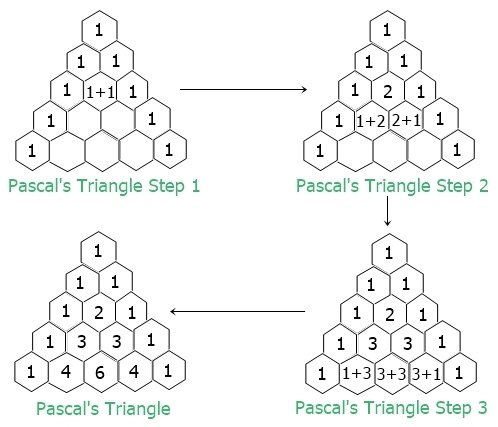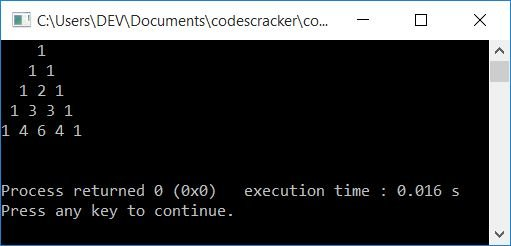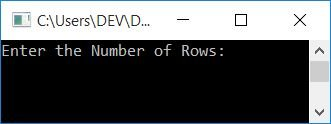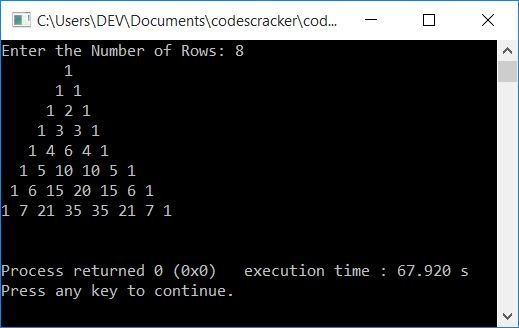# C++ Program to Print Pascal's Triangle

In this article, you will learn and get code to print Pascal's triangle using a C++ program. Here is the list of programs for printing Pascal's triangle:

Before creating these programs, let's understand how  Pascal's triangle gets created.

### What is Pascal's Triangle?

The figure given below shows everything about Pascal's triangle:• How it gets expanded
• What is its structure
• What is the formula to find the value of any column in any row

or whatever you want to learn about it, use Pascal's Triangle to get all the necessary information.

## Print Pascal's Triangle in C++

This program prints Pascal's triangle of five rows or lines. This program doesn't allow the user to define the size of Pascal's triangle. Later on, the same program is created that receives input from the user to define the size of Pascal's triangle.

```#include<iostream>
using namespace std;
int main()
{
int row, col, i=1, j=0, arr, arrTemp;
arr = 1;
arr = 1;
for(row=0; row<5; row++)
{
for(col=4; col>row; col--)
cout<<" ";
for(col=0; col<=row; col++)
{
if(row==0)
cout<<"1";
else
{
if(col==0 || col==row)
cout<<"1 ";
else
{
arrTemp[i] = arr[j]+arr[j+1];
cout<<arrTemp[i]<<" ";
i++;
j++;
}
}
}
cout<<endl;
arrTemp[i] = 1;
if(row>1)
{
j=0;
arr[j]=1;
for(j=1, i=1; j<=row; j++, i++)
arr[j] = arrTemp[i];
i=1;
j=0;
}
}
cout<<endl;
return 0;
}```

This program was built and runs under the Code::Blocks IDE. Here is its sample output:## Print Pascal's Triangle using a Function and Formula in C++

Now let's create another program that does the same job as the previous program. That is, this program also prints Pascal's triangle. The only difference is that here we've used a user-defined function, fact(), that is used to return the factorial of a number passed as its argument, and a formula to find the column's value for every row:

```#include<iostream>
using namespace std;
long int fact(int);
int main()
{
int i, c;
for(i=0; i<5; i++)
{
for(c=4; c>i; c--)
cout<<" ";
for(c=0; c<=i; c++)
cout<<fact(i)/(fact(c)*fact(i-c))<<" ";
cout<<endl;
}
cout<<endl;
return 0;
}
long int fact(int n)
{
int i, f=1;
for(i=1; i<=n; i++)
f = f*i;
return f;
}```

This program produces the same output as the previous program. The formula used in the above program to find the column's value for every row is:

`value = (row!)/((column!)*(row-columns)!)`

Note: The asterisk (!) indicates factorial.

Take note that both the row and the column begin at 0. To find the value in the second column of the fourth row, for example, here is the step-by-step calculation of the value using the above formula:

```value = (row!)/((column!)*(row-columns)!)
= (4!)/((2!)*(4-2)!)
= (24)/(2*(2!))
= 24/(2*2)
= 24/4
= 6```

So 6 is the value that has to be present in the fourth row and second column.

## Print Pascal's Triangle up to n rows in C++

This is the last program on the printing of Pascal's Triangle. This program allows the user to define the size of Pascal's triangle. That is, up to how many rows the user wants to expand Pascal's triangle.

```#include<iostream>
using namespace std;
long int fact(int);
int main()
{
int i, c, rowSize;
cout<<"Enter the Number of Rows: ";
cin>>rowSize;
for(i=0; i<rowSize; i++)
{
for(c=(rowSize-1); c>i; c--)
cout<<" ";
for(c=0; c<=i; c++)
cout<<fact(i)/(fact(c)*fact(i-c))<<" ";
cout<<endl;
}
cout<<endl;
return 0;
}
long int fact(int n)
{
int i, factRes=1;
for(i=1; i<=n; i++)
factRes = factRes*i;
return factRes;
}```

Here is the initial output of this program's sample run:Now supply the input as the number of rows to print Pascal's triangle. Here is an example output with the row size set to 8 by the user:#### The same program in different languages

C++ Quiz

« Previous Program Next Program »